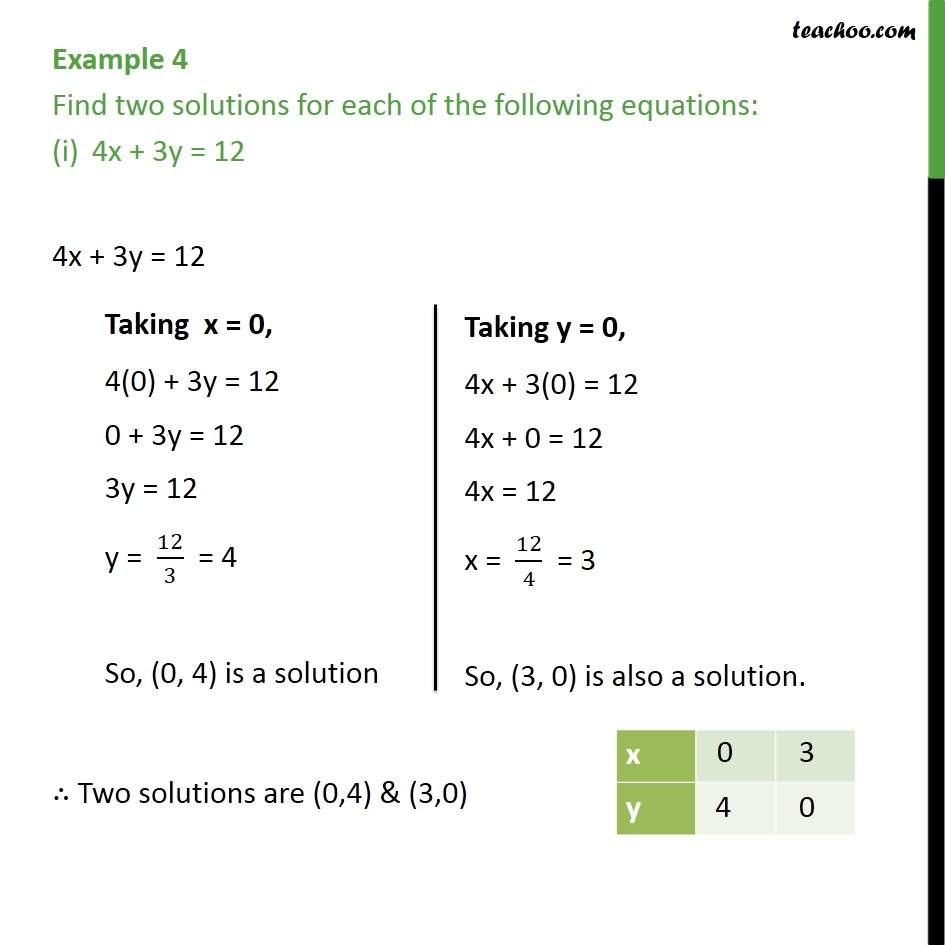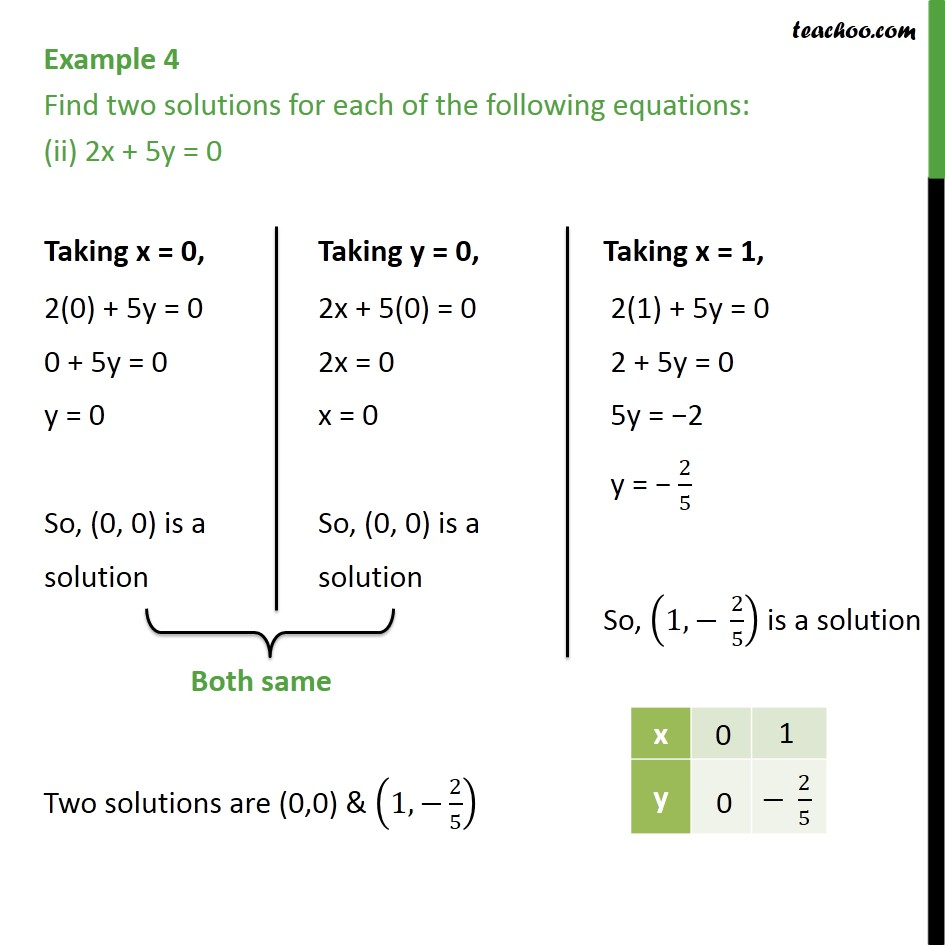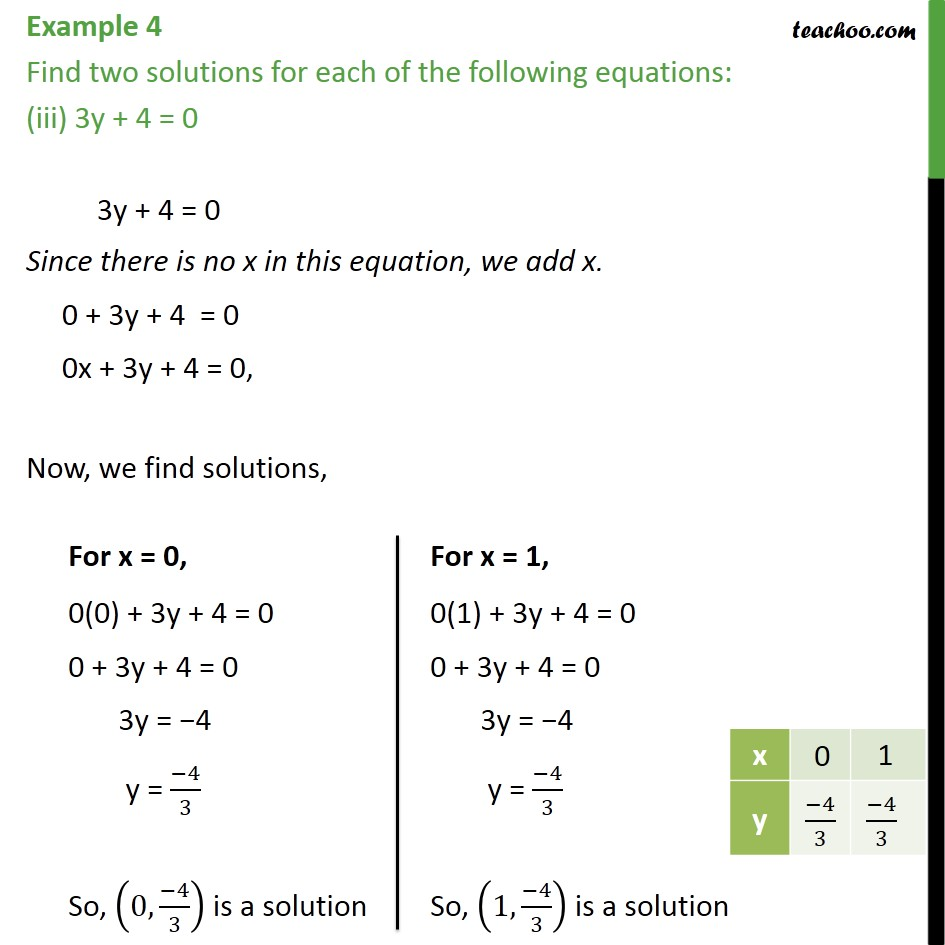Subscribe to our Youtube Channel - https://you.tube/teachoo

1. Chapter 4 Class 9 Linear Equations in Two Variables
2. Concept wise
3. Solution of linear equation

Transcript

Example 4 Find two solutions for each of the following equations: 4x + 3y = 12 4x + 3y = 12 Two solutions are (0,4) & (3,0) Example 4 Find two solutions for each of the following equations: (ii) 2x + 5y = 0 Two solutions are (0,0) & (1, 2/5) Example 4 Find two solutions for each of the following equations: (iii) 3y + 4 = 0 3y + 4 = 0 Since there is no x in this equation, we add x. 0 + 3y + 4 = 0 0x + 3y + 4 = 0, Now, we find solutions,

Solution of linear equation